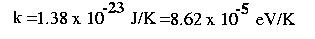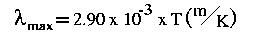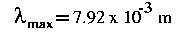QUESTION #210

# What is meant when one says that the temperature of the cosmic background radiation is 3K?

Any object with internal energy (a temperature above absolute zero, or 0 K) radiates that energy in the form of electromagnetic waves (light). The theoretical distribution of wavelengths in that radiation represents 'black body' radiation, and is described mathematically by an equation called Planck's Law. Plotting intensity vs. wavelength, the resulting curve peaks at a wavelength that depends on temperature--the higher the temperature, the shorter its peak wavelength will be. That consequence of Planck's equation is also known as Wien's Law. Also, intensities increase across all wavelengths as temperature increases.

You can see this behavior as an iron rod is heated from room temperature. At first, all of the radiation is in the infrared region, which has a wavelength too long for humans to see. As the rod's temperature increases, it glows red as emitted wavelengths decrease into the visible range. Next, you see orange, then yellow, then white as peak intensity moves to shorter and shorter wavelengths. By analyzing the spectrum of wavelengths, temperature can be calculated. Temperatures of astronomical objects can be determined with this technique. Extreme temperatures near a black hole, for example, peak in the region of very short X-Rays. Stars like our Sun emit primarily in the visible region, and cooler objects like planets emit invisible infrared radiation.

The extremely high temperature of the Big Bang released intense, very short wavelength radiation, but the subsequent cooling of the universe has shifted those wavelengths to the microwave region. (This can also be interpreted as the stretching of wavelengths along with the expansion of the Universe.) Because microwaves have wavelengths longer than even invisible infrared radiation, they are observed in the radio region of the spectrum with radio telescopes. The remnant 'background radiation' from the Big Bang is now seen coming from all directions in the Universe. If you plot this radiation's intensity for different wavelengths, it matches the curve for a temperature of between 2K and 3K.
Answered by: Paul Walorski, B.A. Physics, Part-time Physics Instructor

The short answer is that experimentalists measure the wavelength of the cosmic microwave background photons (and as you might guess by the name they tend to have microwave wavelengths). This can be converted to a photon energy via the relation:and from there, you convert to an equivalent temperature by using the Boltzmann constantSo when a cosmologist talks about the 'temperature' of a photon they are basically describing the equivalent energy of a photon.

As to why temperature is a useful variable, it is important to point out that not every photon from the cosmic background has a temperature of 2.7K. In fact, there is a whole range of energies (or temperatures). However, this range is precisely the spectrum you'd expect for a blackbody radiating energy at a temperature of 2.7K. If you use the Wien displacement lawto find the peak in the spectrum for a blackbody at a temperature of 2.7K you find the peak wavelength isand this peak wavelength is precisely what the experimentalists observe in the photon spectrum of the cosmic background radiation.

So it's not so much that the photons are all at a temperature of 2.7K but rather that they appear as if they were emitted by a single blackbody which was itself at a temperature of 2.7K.
Answered by: Brent Nelson, M.A., Physics Ph.D. student, UC Berkeley